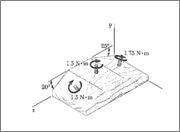Homework Statement

Replace the three couples with a single resultant couple. Specify its magnitude and direction of its axis using angles to the positive x, y, and z axes.Homework Equations

M = r x F (r cross F)

The Attempt at a Solution

I'm pretty sure I want to resolve the moments into their force components, but to do that I would need to set an r. But since I have no measurements other than the angle of inclines I don't see how I can do that.

PhanthomJay
Homework Helper
Gold Member

Homework Statement

Replace the three couples with a single resultant couple. Specify its magnitude and direction of its axis using angles to the positive x, y, and z axes.Homework Equations

M = r x F (r cross F)

The Attempt at a Solution

I'm pretty sure I want to resolve the moments into their force components, but to do that I would need to set an r. But since I have no measurements other than the angle of inclines I don't see how I can do that.
The nice thing about couples is that they are independent of 'r'....the moment of a couple about any point is the couple itself. The couple can be represented by a vector pointing perpendicular to the plane of the couple, following the right hand rule method for its direction (+ or - ) perpendicular to the plane. Then solve the resultant couple as a vector located anywhere on the plane, but with a certain magnitude and direction.

Then I guess this question isn't that complicated at all. If you number the couples 1-3 going from left to right:
M1x=0
M1y=1.5cos20
M1z=1.5sin20

M2x=0
M2y=1.5
M2z=0

M3x=0
M3y=1.75cos25
M3y=-1.75sin25

Therefore MRx=0
MRy=4.496
MRz=-0.2265

MR has a magnitude of 4.5012

And the angles are 0 with the +x axis, 2.91 with the +y axis, and 87.1 with the -z axis (using simple trig)

Barring any issues with significant digits, I think that makes some sense since the resultant is almost vertical.

PhanthomJay